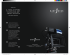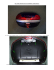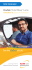# 1 - 3B Scientific

## Transcription

1 - 3B Scientific
```O p t i c s / G eo m e t r i c op t i c s
UE4010100
Lens equation
UE4010100
B a s ic P RINCIP L E S
E VA L U ATION
The focal length f of a lens refers to the distance between the main
plane of the lens and its focal point, see Fig.1. This can be determined
using the Bessel method (devised by Friedrich Wilhelm Bessel). This
involves measuring the various separations between the optical components on the optical bench.
A formula for the focal length of a thin lens can be derived using the
Bessel method from equation (4) f =
a2 − e 2
4a
From Fig.1 and Fig. 2 it can be seen that the following relationship must
apply for a thin lens:
f
(1)
f
a = b+ g
a: distance between object G and image B
b: distance between lens and image B
g: distance between object G and lens
By plugging these values into the lens equation
1 1 1
= +
f b g
(2)
f: focal length of lens
E X P E RIME N T
P R O CE DURE
OBJECTIVE
the following is obtained:
Determine the focal length of a lens using the Bessel method
(3)
• Determine the two positions of a thin
lens where a sharp image is formed.
• Determine the focal length of a thin
lens.
Fig. 1: Schematic showing the definition of focal length for a thin lens
a
1
=
f a⋅ g − g2
g
This corresponds to a quadratic equation with the following pair of solutions:
S UMMA R Y
On an optical bench it is possible to set up a light source, a lens, a screen and an object to be imaged
in such a way that a well focussed image appears on the screen. Using the geometric relationships
between the ray paths for a thin lens, it is possible to determine its focal length.
(4)
g1,2 =
b
f
f
G
B
a
a2
±
− a⋅ f .
4
2
A sharp image is obtained for each of the object distances g1 and g2. The
difference e between them allows the focal length to be determined:
Fig.2: Schematic of ray paths through a lens
a=g+b
Re q uire d Appa r at us
Quantity Description
(5)
Number
1
Optical Bench K, 1000 mm
1009696
4
Optical Rider K
1000862
1
Optical Lamp K
1000863
1
Transformer 12 V, 25 VA (230 V, 50/60 Hz)
1000866or
Transformer 12 V, 25 VA (115 V, 50/60 Hz)
1000865
Convex Lens K, f = 50 mm
1000869
1
1
Convex Lens K, f = 100 mm
1010300
1
Clamp K
1008518
1
Set of 4 Image Objects
1000886
1
Projection Screen K, White
1000879
e
e = g1 − g 2 = a − 4af
2
The difference e is the difference between the two lens positions P1 and P2,
which result in a focussed image.
P1
G
B
S1
S2
Fig.3: Schematic showing the two lens positions which result in a well
focussed image on the screen
1
3B Scientific® Experiments
P2
...going one step further
```

### The author has no financial interest to disclose. How Many of your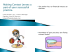### ARRI Anamorphic Ultra Wide Zoom Lens AUWZ 19-36/T4.2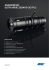### LS-100/LS-200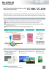### Canon 60D camera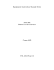### Nivo 2x2 Instructions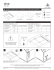### Portatile e senza fili, per uno screening di qualità Portatile e senza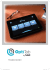### Spec Sheet - N-Vision Optics PVS-7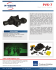### Easyfocus GmbH Pummergasse 20 | A 3002 Purkersdorf | Austria